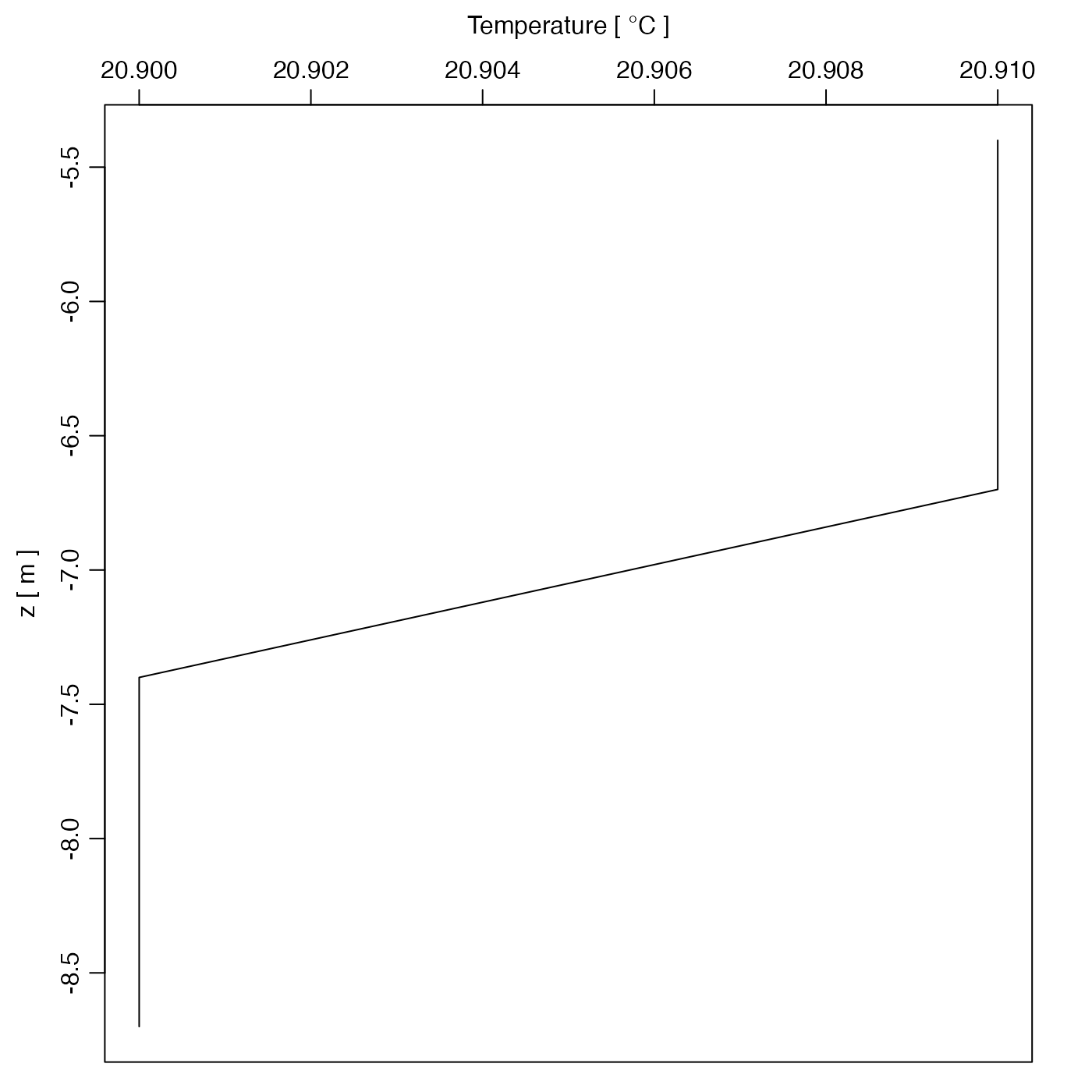Plots data contained in an xbt object.

# S4 method for xbt
plot(
x,
which = 1,
type = "l",
mgp = getOption("oceMgp"),
mar,
debug = getOption("oceDebug"),
...
)

## Arguments

x an xbt object. list of desired plot types; see “Details” for the meanings of various values of which. type of plot, as for plot(). 3-element numerical vector to use for par(mgp), and also for par(mar), computed from this. The default is tighter than the R default, in order to use more space for the data and less for the axes. value to be used with par("mar"). a flag that turns on debugging. Set to 1 to get a moderate amount of debugging information, or to 2 to get more. optional arguments passed to plotting functions.

## Details

The panels are controlled by the which argument, with choices as follows.

• which=1 for a temperature profile as a function of depth.

• which=2 for a soundSpeed profile as a function of depth.

Other functions that plot oce data: plot,adp-method, plot,adv-method, plot,amsr-method, plot,argo-method, plot,bremen-method, plot,cm-method, plot,coastline-method, plot,ctd-method, plot,gps-method, plot,ladp-method, plot,landsat-method, plot,lisst-method, plot,lobo-method, plot,met-method, plot,odf-method, plot,rsk-method, plot,satellite-method, plot,sealevel-method, plot,section-method, plot,tidem-method, plot,topo-method, plot,windrose-method, plotProfile(), plotScan(), plotTS(), tidem-class

Other things related to xbt data: [[,xbt-method, [[<-,xbt-method, as.xbt(), read.xbt.noaa1(), read.xbt(), subset,xbt-method, summary,xbt-method, xbt-class, xbt.edf, xbt

## Examples

library(oce)
data(xbt)
summary(xbt)#> xbt summary
#> -----------
#>
#> * File source:        "/Users/kelley/git/oce/create_data/xbt/xbt.edf"
#> * Serial Number:      0
#> * Longitude:          4.005
#> * Latitude:           -4
#> * Time:               2000-10-10 08:49:38
#> * Data Overview
#>
#>                                Min.   Mean   Max. Dim. NAs   OriginalName
#>     depth [m]                   5.4 7.0333    8.7    6   0          Depth
#>     temperature [°C, ITS-90]   20.9 20.905  20.91    6   0    Temperature
#>     soundSpeed [m/s]         1575.3 1575.3 1575.4    6   0 Sound Velocity
#>
#> * Processing Log
#>
#>     - 2020-02-24 15:07:55 UTC: create 'xbt' object
#>     - 2020-02-24 15:07:55 UTC: read.xbt.edf(file = file, debug = 10)plot(xbt)